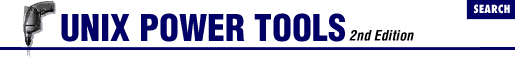home | O'Reilly's CD bookshelfs | FreeBSD | Linux | Cisco | Cisco Exam

#Chapter 5Setting Up Your Terminal## 5.4 Setting the TERMCAP Variable with tset

Warning! For C shell users, tset (5.3 ) has an even more powerful function. The -s option causes it to send to standard output a series of C shell commands not only to set TERM , but also to set the TERMCAP variable to the actual contents of the termcap entry. This speeds up launch time for programs that use termcap : they no longer need to search through the /etc/termcap file until they find the relevant entry; it is already at hand.

Invoke tset as follows:

``` eval ``` ```set noglob eval `tset -Q -s ```other-options-here ``` ````

In order to understand what tset is doing, let's take a moment to send its output to the screen (i.e., issue the command without evaluating it):

```% ```tset -Q -s wy50
```

set noglob;
setenv TERM wy50 ;
setenv TERMCAP 'n9|wy50:li#24:co#80:am:bs:bw:ul:\
:cm=\E=%+\040%+\040:nd=^L:up=^K:do=^J:ho=^^:bt=\EI:\
:cl=^Z:ce=\ET:cd=\EY:al=\EE:dl=\ER:ic=\EQ:dc=\EW:\
:so=\EG4:se=\EGO:sg#1:ue=\EGO:us=\EG8:ug#1\040:\
:me=\E(EGO:mb=\EG2:mp=\E):mh=\EGp:mr=\EG4:mk=\EG1:\
:kl=^H:kr=^L:ku=^K:kd=^J:kh=^^:k1=^A@^M:k2=^AA^M:\
(Article 41.11 explains the format of termcap entries.) The `set`  `noglob` command (6.9 ) causes the shell to suspend interpretation of special characters; the presence of these characters in the termcap entry could cause problems. After execution, the shell is reset to its normal state.5.3 Setting the Terminal Type When You Log In5.5 Querying Your Terminal Type: qterm